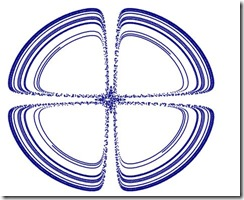# 用Excel展演浑沌！

Excel展演浑沌！

x_(n+1)=sin(by_n)+csin(bx_n),

y_(n+1)=sin(ax_n)+dsin(ay_n),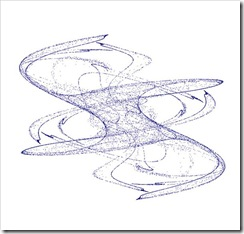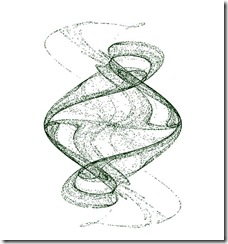2006年4月28日

#### 用Excel展演浑沌增补：变形金刚&三翅鹰

##### 星定 发表于 2006-04-28 20:48:55

———————————————————————————————

Xn+1=μXn（1－Xn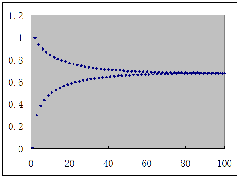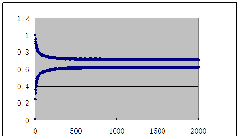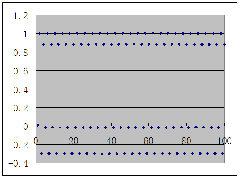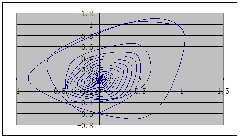Xn+1=1-aXn2+Yn，Yn+1=bXn，X1=Y1=1，a=0.1，b=1生成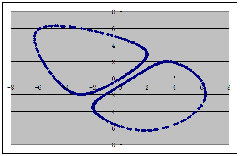Xn+1=1-aXn2+Yn，Yn+1=bXn，X1=Y1=0.5，a=0.134，b=1生成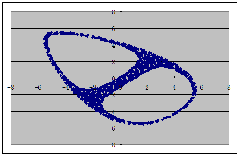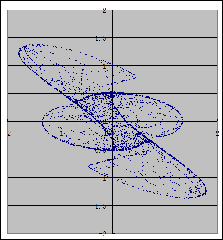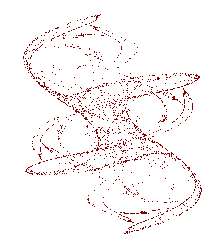Xn+1=sin(aYn)-zcos(bX n),

Yn+1=zsin(cXn)-cos(dY n),

Zn+1=esinXn.#### 用Excel展演浑沌！（加强版）

x_(n+1)=sin(by_n)+csin(bx_n),

y_(n+1)=sin(ax_n)+dsin(ay_n),

2006年4月28日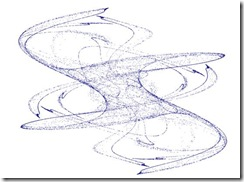x_(n+1)=(x_n+Ksiny_n)cos(2π/q)+y_nsin(2π/q)

y_(n+1)=-(x_n+Ksiny_n)sin(2π/q)+y_ncos(2π/q)

q为3至6的整数。1，1，-0.6，0.95，=2-2*C1，=C1*A1+E1*A1*A1/(1+A1*A1)，=A1

=D1*B1+F1，=F2-G1，=C1，=D1，=2-2*C2，=C1*A2+E1*H2/(1+H2)，=A2，=A2*A2